# 5.4.5 - Linear Regression Reports - Teradata Warehouse Miner

## Teradata Warehouse Miner User Guide - Volume 3Analytic Functions

Product
Release Number
5.4.5
Published
February 2018
Language
English (United States)
Last Update
2018-05-04
dita:mapPath
yuy1504291362546.ditamap
dita:ditavalPath
ft:empty
dita:id
tbj1499730075274

## Data Quality Reports

• Variable Statistics — If selected on the Results Options tab, this report gives the mean value and standard deviation of each variable in the model based on the SSCP matrix provided as input.
• Near Dependency — If selected on the Results Options tab, this report lists collinear variables or near dependencies in the data based on the SSCP matrix provided as input. Entries in the Near Dependency report are triggered by two conditions occurring simultaneously. The first is the occurrence of a large condition index value associated with a specially constructed principal factor. If a factor has a condition index greater than the parameter specified on the Results Option tab, it is a candidate for the Near Dependency report. The other is when two or more variables have a variance proportion greater than a threshold value for a factor with a high condition index. Another way of saying this is that a ‘suspect’ factor accounts for a high proportion of the variance of two or more variables. The parameter to defines what a high proportion of variance is also set on the Results Option tab. A default value of 0.5.
• Detailed Collinearity Diagnostics — If selected on the Results Options tab, this report provides the details behind the Near Dependency report, consisting of the following tables.
• Eigenvalues of Unit Scaled X'X — Report of the eigenvalues of all variables scaled so that each variable adds up to 1 when summed over all the observations or rows. In order to calculate the singular values of X (the rows of X are the observations), the mathematically equivalent square root of the eigenvalues of XTX are computed instead for practical reasons.
• Condition Indices — The condition index of each eigenvalue, calculated as the square root of the ratio of the largest eigenvalue to the given eigenvalue, a value always 1 or greater.
• Variance Proportions — The variance decomposition of these eigenvalues is computed using the eigenvalues together with the eigenvectors associated with them. The result is a matrix giving, for each variable, the proportion of variance associated with each eigenvalue.

## Linear Regression Step N (Stepwise-only)

• Linear Regression Model Assessment
• Squared Multiple Correlation Coefficient (R-squared) — This is the same value calculated for the Linear Regression report, but it is calculated here for the model as it stands at this step. The closer to 1 its value is, the more effective the model.
• Standard Error of Estimate — This is the same value calculated for the Linear Regression report, but it is calculated here for the model as it stands at this step.
• In Report — This report contains the same fields as the Variables in Model report (described below) with the addition of the following field.
• F Stat — F Stat is the partial F statistic for this variable in the model, which may be used to decide its inclusion in the model.

A quantity called the extra sums of squares is first calculated as follows: ESS = “DRS with x” - “DRS w/o”, where DRS is the Regression Sums of squares or “due-to-regression sums of squares”.

Then the partial F statistic is given by F(xi) = ESS(xi) / meanRSS(xi), where meanRSS is the Residual Mean Square.

• Out Report
• Independent Variable — This is an independent variable not included in the model at this step.
• P-Value — This is the probability associated with the T-statistic associated with each variable not in, or excluded from, the model, as described for the Variables in Model report as T Stat and P-Value. (Note that it is not the P-Value associated with F Stat).

When the P-Value is used for step decisions, a forward step consists of adding the variable with the smallest P-value providing it is less than the criterion to enter. For backward steps, all the probabilities or P-values are calculated for the variables currently in the model at one time, and the one with the largest P-value is removed if it is greater than the criterion to remove.

• F Stat — F Stat is the partial F statistic for this variable in the model, which may be used to decide its inclusion in the model.

A quantity called the extra sums of squares is first calculated as follows: ESS = “DRS with xi” - “DRS w/o xi”, where DRS is the Regression Sums of squares or “due-to-regression sums of squares”.

Then the partial F statistic is given by F(xi) = ESS(xi) / meanRSS(xi), where meanRSS is the Residual Mean Square.

• Partial Correlation — The partial correlation coefficient for a variable not in the model is based on the square root of a measure called the coefficient of partial determination, which represents the marginal contribution of the variable to a model that does not include the variable. Here, contribution to the model means reduction in the unexplained variation of the dependent variable.
The formula for the partial correlation of the i th independent variable in the linear regression model built from all the independent variables is given by: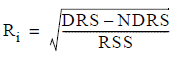where the following is true:

DRS is the Regression Sums of squares for the model including those variables currently in the model, NDRS is the Regression Sums of squares for the current model without the i th variable

RSS is the Residual Sums of squares for the current model.

## Linear Regression Model

• Total Observations — This is the number of rows originally summarized in the SSCP matrix that the linear regression analysis is based on. The number of observations reflects the row count after any rows were eliminated by listwise deletion (recommended) when the matrix was built.
• Total Sums of squares — The so-called Total Sums of squares is given by the equation TSS = ∑(y –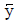)2 where y is the dependent variable that is being predicted andis its mean value. The Total Sums of squares is sometimes also called the total sums of squares about the mean. Of particular interest is its relation to the “due-to-regression sums of squares” and the “residual sums of squares” given by TSS = DRS + RSS. This is a shorthand form of what is sometimes known as the fundamental equation of regression analysis: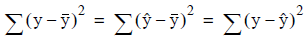where y is the dependent variable,is its mean value, and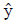is its predicted value.

• Multiple Correlation Coefficient (R) — The multiple correlation coefficient R is the correlation between the real dependent variable y values and the values predicted based on the independent x variables, sometimes written Ry · x1x2...xn , which is calculated in Teradata Warehouse Miner simply as the positive square root of the Squared Multiple Correlation Coefficient (R 2 ) value.
• Squared Multiple Correlation Coefficient (R-squared) — The squared multiple correlation coefficient R 2 is a measure of the improvement of the fit given by the linear regression model over estimating the dependent variable y naïvely with the mean value of y. It is given by: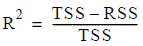where TSS is the Total Sums of squares and RSS is the Residual Sums of squares. It has a value between 0 and 1, with 1 indicating the maximum improvement in fit over estimating y naïvely with the mean value of y.

• Adjusted R-squared — The adjusted R 2 value is a variation of the Squared Multiple Correlation Coefficient (R 2 ) that has been adjusted for the number of observations and independent variables in the model. Its formula is given by: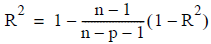where n is the number of observations and p is the number of independent variables (substitute n-p in the denominator if there is no constant term).

• Standard Error of Estimate — The standard error of estimate is calculated as the square root of the average squared residual value over all the observations, i.e.,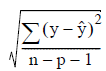where y is the actual value of the dependent variable,is its predicted value, n is the number of observations, and p is the number of independent variables (substitute n-p in the denominator if there is no constant term).

• Regression Sums of squares — This is the “due-to-regression sums of squares” or DRS referred to in the description of the Total Sums of squares, where it is pointed out that TSS = DRS + RSS. It is also the middle term in what is sometimes known as the fundamental equation of regression analysis: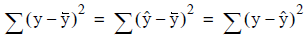where y is the dependent variable,is its mean value andis its predicted value.

• Regression Degrees of Freedom — The Regression Degrees of Freedom is equal to the number of independent variables in the linear regression model. It is used in the calculation of the Regression Mean-Square.
• Regression Mean-Square — The Regression Mean-Square is simply the Regression Sums of squares divided by the Regression Degrees of Freedom. This value is also the numerator in the calculation of the Regression F Ratio.
• Regression F Ratio — A statistical test called an F-test is made to determine if all the independent x variables taken together explain a statistically significant amount of variation in the dependent variable y. This test is carried out on the F-ratio given by: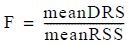where the following is true:
• meanDRS is the Regression Mean-Square
• meanRSS is the Residual Mean-Square.

A large value of the F Ratio means that the model as a whole is statistically significant.

The easiest way to assess the significance of this term in the model is to check if the associated Regression P-Value is less than 0.05. However, the critical value of the F Ratio could be looked up in an F distribution table. This value is very roughly in the range of 1 to 3, depending on the number of observations and variables.

• Regression P-value — This is the probability or P-value associated with the statistical test on the Regression F Ratio. This statistical F-test is made to determine if all the independent x variables taken together explain a statistically significant amount of variation in the dependent variable y. A value close to 0 indicates that they do.

The hypothesis being tested or null hypothesis is that the coefficients in the model are all zero except the constant term (i.e., all the corresponding independent variables together contribute nothing to the model). The P-value in this case is the probability that the null hypothesis is true and the given F statistic has the value it has or smaller. A right tail test on the F distribution is performed with a 5% significance level used by convention. If the P-value is less than the significance level (i.e., less than 0.05), the null hypothesis should be rejected (i.e., the coefficients taken together are significant and not all 0).

• Residual Sums of squares — The residual sums of squares or sum of squared errors RSS is simply the sum of the squared differences between the dependent variable estimated by the model and the actual value of y, over all of the rows: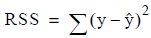• Residual Degrees of Freedom — The Residual Degrees of Freedom is given by n-p-1 where the following is true:
• n is the number of observations
• p is the number of independent variables (or n-p if there is no constant term). It is used in the calculation of the Residual Mean-Square.
• Residual Mean-Square — The Residual Mean-Square is simply the Residual Sums of squares divided by the Residual Degrees of Freedom. This value is also the denominator in the calculation of the Regression F Ratio.

## Linear Regression Variables in Model Report

• Dependent Variable — The dependent variable is the variable being predicted by the linear regression model.
• Independent Variable — Each independent variable in the model is listed along with accompanying measures. Unless the user deselects the option Include Constant on the Regression Options tab of the input dialog, the first independent variable listed is CONSTANT, a fixed value representing the constant term in the linear regression model.
• B Coefficient — Linear regression attempts to find the b-coefficients in the equation= b0 + b1x1 + bnxn in order to best predict the value of the dependent variable y based on the independent variables x1 to xn. The best values of the coefficients are defined to be the values that minimize the sum of squared error values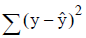over all the observations.

• Standard Error — This is the standard error of the B Coefficient term of the linear regression model, a measure of how accurate the B Coefficient term is over all the observations used to build the model. It is the basis for estimating a confidence interval for the B Coefficient value.
• T Statistic — The T-statistic is the ratio of a B Coefficient value to its standard error (Std Error). Along with the associated t-distribution probability value or P-value, it can be used to assess the statistical significance of this term in the linear model.

The easiest way to assess the significance of this term in the model is to check if the P-value is less than 0.05. However, one could look up the critical T Stat value in a two-tailed T distribution table with probability .95 and degrees of freedom roughly the number of observations minus the number of variables. This would show that for all practical purposes, if the absolute value of T Stat is greater than 2 the model term is statistically significant.

• P-value — This is the t-distribution probability value associated with the T-statistic (T Stat), that is, the ratio of the b-coefficient value to its standard error (Std Error). It can be used to assess the statistical significance of this term in the linear model. A value close to 0 implies statistical significance and means this term in the model is important.

The hypothesis being tested or null hypothesis is that the coefficient in the model is actually zero (i.e., the corresponding independent variable contributes nothing to the model). The P-value in this case is the probability that the null hypothesis is true and the given T-statistic has the absolute value it has or smaller. A two-tailed test on the t-distribution is performed with a 5% significance level used by convention. If the P-value is less than the significance level (i.e., less than 0.05), the null hypothesis should be rejected (i.e., the coefficient is statistically significant and not 0).

• Squared Multiple Correlation Coefficient (R-squared) — The Squared Multiple Correlation Coefficient (Rk 2) is a measure of the correlation of this, the k th variable with respect to the other independent variables in the model taken together. (This measure should not be confused with the R2 measure of the same name that applies to the model taken as a whole). The value ranges from 0 to 1 with 0 indicating a lack of correlation and 1 indicating the maximum correlation. It is not calculated for the constant term in the model.
Multiple correlation coefficients are sometimes presented in related forms such as variance inflation factors or tolerances. The variance inflation factor is given by the formula: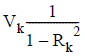where V k is the variance inflation factor and R k 2 is the squared multiple correlation coefficient for the k th independent variable. Tolerance is given by the formula Tk = 1 – Rk 2 where T k is the tolerance of the k th independent variable and R k 2 is as before.

Refer to Multiple Correlation Coefficients for details on the limitations of using this measure to detect collinearity problems in the data.

• Lower — Lower is the lower value in the confidence interval for this coefficient and is based on its standard error value. For example, if the coefficient has a value of 6 and a confidence interval of 5 to 7, it means that according to the normal error distribution assumptions of the model, there is a 95% probability that the true population value of the coefficient is actually between 5 and 7.
• Upper — Upper is the upper value in the confidence interval for this coefficient based on its standard error value. For example, if the coefficient has a value of 6 and a confidence interval of 5 to 7, it means that according to the normal error distribution assumptions of the model, there is a 95% probability that the true population value of the coefficient is actually between 5 and 7.
• Standard Coefficient — Standardized coefficients, sometimes called beta-coefficients, express the linear model in terms of the z-scores or standardized values of the independent variables. Standardized values cast each variable into units measuring the number of standard deviations away from the mean value for that variable. The advantage of examining standardized coefficients is that they are scaled equivalently, so that their relative importance in the model can be more easily seen.
• Incremental R-squared — It is possible to calculate the model’s Squared Multiple Correlation value incrementally by considering the cumulative contributions of x variables added to the model one at a time, namely Ry · x1 , Ry ·x1x2 , …, Ry · x1x2...xn . These are called Incremental R 2 values, and they give a measure of how much the addition of each x variable contributes to explaining the variation in y in the observations.